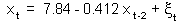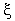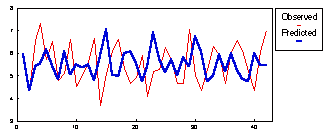## 4.5. Stochastic models based on regression

Our objective is to reproduce the pattern of population change rather than to predict the most probable population counts in the next year. Our model for the fox could not predict the pattern of population change: predicted density approached a steady state by damped oscillations, whereas in nature there are quasi-periodic cycles.

This model can reproduce the pattern of population change better if stochastic noise is added which can draw simulated population counts away from the steady state. Noise can be simulated by a random variable which distribution is normal with zero mean. Zero mean is important as a non-biasedness condition. The variance (or SD which is the square root of the variance) should be set equal to the error variance: [SSE/df(SSE)] of regression.

Stochastic version of the fox population model is:Random variablehas a normal distribution with zero mean and variance equal to the error variance in regression: Var() = 0.26 (S.D.= 0.51).

Stochastic model generates quasi-periodic cycles similar to that in the real population:Alexei Sharov 2/03/97